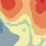# CROSS-VARIOGRAM NEGATIVE -COKRING

358
4
Jump to solution
09-06-2019 06:45 PMNew Contributor

Dear all,

I am a junior researcher and not fully experience in Geostatistical Analyst, so I hope someone can help me with the following.

How do I interpret a negative cross-variogram (Figure)? I can't understand the parameters, nugget is zero for cross-variogram, is that it? Because I have the nugget of Z1, Z2 and I do not have the Z1 x Z2. Only the partial sill.

Thanks a lot,

Ramon

Tags (1)
1 Solution

Accepted SolutionsbyEsri Regular Contributor

There is no nugget for the cross-covariance (it's not wrong to say that nugget = 0).  The Major Range is shared between all three models (the semivariogram for the primary, semivariogram for the secondary, and cross-covariance between them).  The indices for the Partial Sill indicate the model.  Partial Sill  is for the primary dataset, Partial Sill  is for the secondary dataset, and Partial Sill  is for the cross-covariance.

For your model, the cross-covariance is:

Type = Gaussian
Nugget = 0
Range = 150.5006
Partial Sill = -0.001909066

The sill is the partial sill plus the nugget, so for your model, you can just call it the sill instead of the partial sill, since the nugget is 0.

4 RepliesbyEsri Regular Contributor

Hi Ramon,

A negative cross-covariance means that the primary and secondary datasets are negatively spatially correlated.  Kriging assumes that each dataset is positively autocorrelated with itself, but the two datasets are allowed to be negatively correlated with each other.  This means that areas where one dataset has a large value, the other dataset tends to have a small value (and vice versa), and kriging is able to use this information to improve the predicted values of the primary dataset.

-EricNew Contributor

Hi Eric,

Thanks so much for the feedback

I can understand the conceptual part, I can not interpret the cross variogram generated in arcgis, what would be the Nuggut, would be zero? You can take a look at the figure, please.

-RamonbyEsri Regular Contributor

There is no nugget for the cross-covariance (it's not wrong to say that nugget = 0).  The Major Range is shared between all three models (the semivariogram for the primary, semivariogram for the secondary, and cross-covariance between them).  The indices for the Partial Sill indicate the model.  Partial Sill  is for the primary dataset, Partial Sill  is for the secondary dataset, and Partial Sill  is for the cross-covariance.

For your model, the cross-covariance is:

Type = Gaussian
Nugget = 0
Range = 150.5006
Partial Sill = -0.001909066

The sill is the partial sill plus the nugget, so for your model, you can just call it the sill instead of the partial sill, since the nugget is 0.New Contributor

Understood. Thank you very much!

-Ramon